By going through these CBSE Class 12 Chemistry Notes Chapter 6 General Principles and Processes of Isolation of Elements, students can recall all the concepts quickly.

## General Principles and Processes of Isolation of Elements Notes Class 12 Chemistry Chapter 6

The extraction and isolation of an element from its combined form involves various principles of chemistry. Metallurgy is the scientific and technological process of extracting a metal from its ore. The natural materials in which the metals or their compounds occur in the earth are called minerals. The mineral from which the metal is extracted conveniently and economically is called an ore. Ores are usually contaminated with earthly or undesired materials known as Gangue.

The extraction and isolation of metals from their respective ores involve the following major steps :

1. The concentration of the ore
2. Isolation of the metal from its concentrated ore, and
3. Purification of the metal.

Principle Ores of Some Important Metals: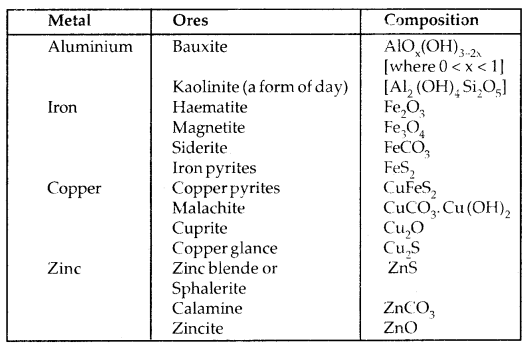Among metals, aluminium is the most abundant. For the purpose of extraction, bauxite is chosen for aluminium. For iron, usually oxide ores [Haematite Fe203] are taken. Before proceeding for concentration, ores are graded and crushed to a reasonable size.

Concentration Of Ores: Removal of the unwanted materials (e.g. sand, clays etc.) from the ore is called concentration, dressing, or benefaction. Unwanted impurities present in the ore are called gangue.

The nature of the impurities, the type of the metal and the environmental factors are taken into consideration.
(a) Hydraulic Washing: it is based on the difference in gravities of the ore and the gangue particles. It is, therefore, a type of gravity separation. When a stream of water is run through the powdered ore, the lighter gangue particles are washed away and heavier ore particles settle down.

(b) Magnetic Separation: This procedure is based on the difference in the magnetic properties of the ore and impurities present in it. When passed over the conveyer belt of a magnetic roller, magnetic particles settle in a heap nearer and non-magnetic impurities a bit away, as shown below: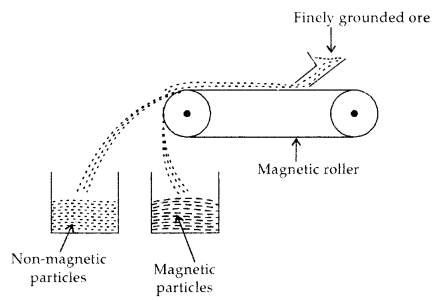Magnetic Separation

Froth Floatation Process: This process is used for removing gangue from sulphide ores only. Powdered sulphide ore is mixed with water to which Collectors (e.g. pine oil, fatty acid, xanthates etc.) enhance the non-wettability of the mineral particles and froth stabilizers (e.g. cresols, aniline) which stabilize the froth ore added. The mineral particles become wet by oils while the gangue particles by water.

A rotating paddle agitates the mixture and draws air in it. As a result, froth is formed which carries the mineral particles. The froth is light and is skimmed off from where ore particles are recovered after drying it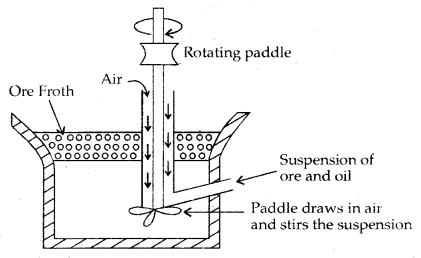Froth Flotation Process

Leaching: This process consists of treating the powdered ore with a suitable reagent (such as acids bases or other chemicals) which can n selectively dissolve the ore but not the impurities.

In Baeyer’s process, pure aluminium oxide is obtained from the bauxite ore (which contains impurities of Fe2Os and silicates) by treating the powdered ore with a 45% solution of NaOH when alumina dissolves leaving behind impurities like Fe203 which are filtered off.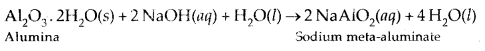Na [Al(OH)4] or NaAlO., is neutralized by CO2 when Al(OH)3 gets precipitated.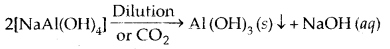Al(OH)3, obtained above is filtered, washed and finally heated to about 1473 K to get pure alumina Al2O3Extraction of Crude Metal From Concentrated Ore: The concentrated ore must be converted into a form that is suitable for a reduction. Sulphide ore is usually converted into its oxide before reduction. Oxides are easier to reduce.

Two steps are involved
(a) Conversion of the concentrated ore into oxide ore
(b) Reduction of the metal oxide into metal

(a) Conversion To Oxide:
1. Calcination: It is the process of converting ore into its oxide by heating it strongly below its melting point either in the absence or limited supply of air. Volatile matter is driven off.2. Roasting: It is the process of converting ore into its metallic oxide by heating strongly at a temperature insufficient to melt in excess of air.
2 ZnS + 3O2 → 2 ZnO + 2 SO2
2 PbS + 3O2 → 2 PbO + 2SO2
2 Cu2S + 3O2 → 2 Cu2O + 2 SO2

(b) Reduction of oxide to the metal: It is done with a suitable reducing agent (C or CO or even another metal)
MxO + yC → xM + yCO

Thermodynamic Principles Of Metallurgy: The change in Gibbs energy ΔG is given by
ΔG = ΔH – TΔS …(1)
where ΔH = Enthalpy change at temperature T
ΔS = Entropy change at temperature T

Also ΔG° = -RT ln K. ……….(2)
where R = Gas constant;
K = Equilibrium constant.
The reaction will proceed if ΔG° is negative K in that case will be positive.

This happens only when the reaction proceeds towards products.
1. When the value of ΔG° is negative in equation (1) then only the reaction will proceed. If ΔS is positive, on increasing the temperature (T), the value of TΔS would increase (ΔH < TΔS) and then ΔG will become -ve.

2. If reactants and products of two reactions are put together in a system and the net ΔG of the possible reactions is – ve, the overall reaction will occur. So the process of interpretation involves the coupling of the two reactions, getting the sum of their ΔG and looking for its magnitude and sign. Such coupling is easily understood through Gibbs energy (ΔG°) vs – T plots for the formation of the oxides.

H.J. T Ellingham gave a graphical representation of Gibbs energy. It provides a sound basis for considering the choice of reducing agent in the reduction of oxides. Such a diagram helps us in predicting the feasibility of the thermal reduction of ore.

The reducing agent forms its oxide when the metal oxide is reduced. The role of the reducing agent is to provide ΔG° negative and large enough to make the sum of ΔG° of the two reactions (oxidation of the reducing agent and reduction of the metal oxide) negative.

As we know, during reduction, the oxide of a metal decomposes.
MxO (s) → xM (Solid or Liquid) + $$\frac{1}{2}$$ O2(g) ……..(3)

The reducing agent takes away the oxygen.
xM (s or l) + $$\frac{1}{2}$$ O2 → MxO(s) ……[ΔG°(M, MxO) ………(4)

If reduction is carried out through equa lion (3), the oxidadion of the reducing agent (e.g. C or CO) will be as:
C(s) + $$\frac{1}{2}$$ Oz(g) → CO(g) …………[ΔG(C, CO)]…..(5)
CO(g) + $$\frac{1}{2}$$ Oz(g) → CO2(g)……[ΔG(C, CO)]…(6)

If carbon is taken, there may be complete oxidation to CO2
$$\frac{1}{2}$$ C(s)+ $$\frac{1}{2}$$O2 (g) → $$\frac{1}{2}$$CO2(g) ….[2 ΔG(C, CO)]…(7)

On subtracting equation (4), we get
MxO(s) + C(s) → xM(s or l) +CO(g) …(8)
MxO (s) + C (s) → xM (s or l) + CO2 (g) …(9)
MxO (s) + $$\frac{1}{2}$$C(s) → xM (s or l) + $$\frac{1}{2}$$CO2 (g). …..(10)

These reactions describe the actual reduction of the metal oxide MxO that is to be accomplished.
Increasing T (Heating) favours a negative value of ΔrG°. Therefore, the temperature is chosen such that the sum of ΔrG° in the two combined redox process is negative.

Applications:
(a) Extraction of Iron from its Oxides: Concentrated oxide ores of iron are mixed with limestone and coke and fed into a Blast furnace from the top. Here coke reduces oxide to the metal as follows:
FeO(s) + C(s) → Fe(s or l) + CO(g) ……….(11)

In two steps:
1. FeO(s) → Fe(s) + $$\frac{1}{2}$$O2 (g) [ΔG(FeO, Fe)] …….(2)
2. C(s) + $$\frac{1}{2}$$ O2 (g) → CO(g) [ΔG(C, CO)] ……..(3)

From (12) and (13), The ne.t Gibbs energy change becomes
ΔG(C, CO) + ΔG(FeO, Fe) = ΔrG ……….(14)

∴ The resultant reaction will take place when the r.h.s. of equation (14) becomes negative.

The reactions occurring in the Blast furnace at different temperatures are as follows:
At 500 – 800 K (lower temp, range)
3 Fe2O3 + CO → 2 Fe3O4 + CO2
Fe3O4 + 4CO → 3 Fe + 4 CO2
Fe2O3 + C0 → 2Fe + CO2

At 900 -1500 K (higher temp, in the blast furnace)
C + CO2 → 2CO
FeO + CO → Fe + CO2

Limestone is also decomposed to CaO which removes silicate impurity of the ore as slag. The slag is in a molten state and separates out from iron.
CaCO3 (s) → CaO (s) + CO2 (g)
CaO (s) + SiO2 (s) → CaSiO (fusible slag)

Iron obtained from Blast furnace contains 4% carbon and many impurities in smaller amount (e.g. S, P, Si, Mn) is called pig iron. Cast iron is different from pig iron. It has a slightly lower carbon content (3%) and is extremely hard and brittle.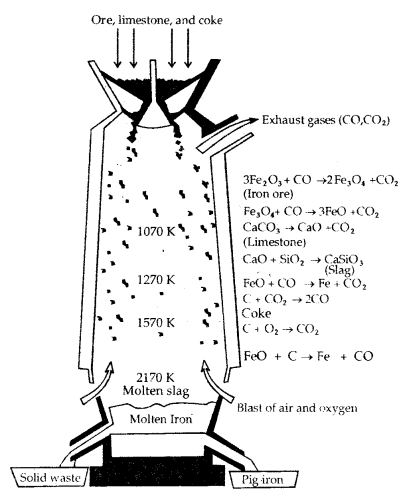Blast Furnace

Further Reductions: Wrought iron or malleable iron is the purest form of commercial iron and is prepared from cast iron by oxidising

impurities in a reverberatory furnace lined with haematite. This haematite oxidises carbon to carbon monoxide :
Fe2O3 + 3 C → 2 Fe + 3CO

Limestone is added as a flux and sulphur, silicon and phosphorus are oxidised and passed into the slag. The metal is removed and freed from the slag by passing through rollers.

(b) Extraction of Copper from Cuprous Oxide [Copper (I) Oxide]: Most of the copper ores are sulphide ores, concentrated sulphide ores are roasted/smelted to give oxides.
2 Cu2S + 3 O2 → 2 Cu2O + S

The oxide can then be easily reduced to give Cu metal.
Cu20 + Ccoke → 2 Cu + CO

In the actual process, the ore is heated in a reverberatory furnace after mixing with silica.
In the furnace, iron oxide slags off’ as iron silicate and copper is produced in the form of copper matte which contains Cu2S and FeS.
FeO + SiO2 → Fe Si O3(slag)

In the silica-lined convertor, copper matte is charged. Some silica is added and a hot air blast is blown when the following reactions take place.
2 FeS + 3O2 → 2 FeO + 2SO2
FeO + SiO2 → FeSiO3
2 Cu2S + 3O2 → 2 Cu2O + 2 SO2
2 Cu2O + Cu2S → 6 Cu + SO2
The solidified copper obtained has a blistered appearance due to the evolution of SO2 and so it is called blister copper.

(C) Extraction of Zinc from Zinc Oxide: Zinc oxide is reduced using cokeThe metal is distilled off and collected by rapid chilling.

Electrochemical Principles Of Metallurgy: In electrolysis, metal ions in solution or molten form are reduced or by adding some reducing element. Here
ΔG° =. – n FE° ….(i)
where n = no. of electrons transferred
E° = Standard e.m.f. of the cell [redox couple]
F = Faraday = 96,500 C
ΔG° = Change in standard Gibbs energy.

More reactive metals have large negative values of the electrode potential. So their reduction is difficult. If the difference of two E° values corresponds to a positive E° and consequently negative ΔG° in the equation
1. above, then the less reactive metal will come out of the solution and more reactive metal will go into the solution.
Cu2+ (aq) + Fe (S) → Cu (S) + Fe2+ (aq)

Examples:
Metallurgy of Aluminium: Purified Al2O3 is mixed with Na3 AlF6 or CaF2 to, lower the melting point of the mix and to make the solution conductive. The fused matrix is electrolysed.
2 Al2O3 + 3C → 4 Al + 3 CO2

This process of electrolysis is widely known as the Hall-Fieroult process.

Steel cathode and graphite anode are used. The graphite anode is useful here for the reduction of oxide to the metal.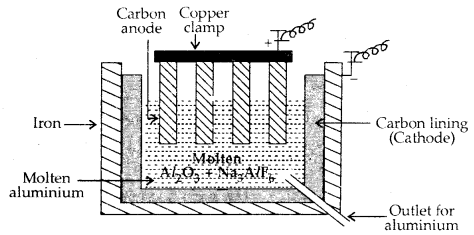Electrolytic cell for the extraction of aluminium

The electrolysis of the molten mass is carried out in an electrolytic cell using carbon electrodes. The oxygen liberated at the anode reacts with the carbon of the anode producing CO and CO2.This way for each kg of aluminium – produced, about 0.5 kg of carbon anode is burnt away.

The electrolytic reactions are:
Cathode: Al3+ (melt) + 3e → Al (l)
Anode: C(s) + O2- (melt) → CO(g) + 2e
C(s) + 2 O2- (melt) → CO2 (g) + 4e

Copper from low-grade ores and scraps: Copper is extracted from low grades ores by Hydrometallurgy. It is leached out using acid or bacteria. The solution containing Cu2+ (aq) is treated with iron or H2.
Cu2+ (aq) + H2 (g) → Cu (s) + 2H+(aq)

Oxidation-Reduction: Some non-metals are extracted based on oxidation. Chlorine from prime solution is oxidized to Cl2.
2 Cl (aq) + 2 H2O (l) → 2 OH (aq) + H2 (g) + Cl2 (g)

In the extraction of gold and silver, metal is leached with CN.
Ag → Ag+ + e oxidation
Au → Au+ + e oxidation

The metal is later recovered by the displacement method.
4 Au (s) + 8 CN (aq) + 2H2O (aq) + O2 → 4 [Au (CN)2] (aq) + 4 OH (aq)
2 [Au (CN)2](aq) + Zn (s) → 2 Au (s) + [Zn (CN)4]2- (aq)
In this reaction zinc acts as a reducing agent.

Refining: To obtain metal of high purity and to remove the last traces of impurities from the extracted metal, they are subjected to refining.

It is based upon the difference in properties of the metal and the impurity.
Several techniques are listed below:

1. Distillation,
2. Liquation,
3. Electrolysis,
4. Zone refining,
5. Vapour phase refining,
6. Chromatographic methods.

1. Distillation: This is useful for low boiling metals like Zn and Hg.

2. Liquation: A low melting metal like tin (Sn) can be made to flow on a sloping surface leaving higher melting impurities.

3. Electrolytic Refining: Impure metal is made anode. A strip of pure metal is made the cathode. The electrolyte (bath) contains soluble salt of the same metal.
Anode: M → Mn+ + e
Cathode: Mn+ + ne → M

Copper is refined by the electrolytic method. Here acidified solution of copper sulphate acts as an electrolyte.
Anode: Cu(s) → Cu2+ + 2e
Cathode: Cu2+ + 2e → Cu(s)

4. Zone Refining: This method is based upon the principle that the impurities are more soluble in the melt than in the solid state of the metal. The molten zone moves along with the mobile heater fixed at one end of the impure metal. As the heater moves forward the pure metal crystallises out of the metal and the impurities pass on into the adjacent molten zone. At one end, impurities get concentrated. This end is cut off.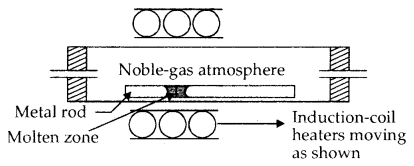Zone-Refining Process

Vapour Phase Refining: Mond’s Process for Nickel RefiningThe carbonyl complex is subjected to a higher temperature so that it is decomposed to give pure metal.van Arkel Method for Refining Zirconium or Titanium: This method is very useful for removing all the oxygen and nitrogen present in the form of impurity in certain metals like Zr and Ti. The crude metal is heated in an evacuated vessel with iodine. The metal iodide, being more covalent, volatilises.
Zr + 2I2 → ZrI4.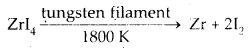Chromatographic Methods: This method is based on the principle that different components of a mixture are differently adsorbed on an adsorbent.

Column Chromatography: It is very useful for the purification of the elements which are available in minute quantities and the impurities are not very different in chemical properties from the element to be purified.

There are several chromatographic techniques such as

1. Paper chromatography
2. Gas chromatography

Uses of Aluminium, Copper, Zinc and Iron: Alloys containing Al are light and are very useful. A1 wires conduct electricity. It is used as a reductant.

Copper is used to making wires in the electrical industry. Several alloys of copper with Zn, Sn and Ni are largely used.
Zinc is used for galvanising iron. It is used as a reducing agent. Similarly different forms of iron: Cast iron, Wrought iron, Steel find wide applications.

A Summary of the Occurrence and Extraction of Some Metals is Presented in the following Table: